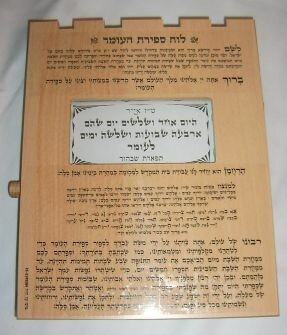## Sefirat haOmerA

### David Bar-TzurWE PRAISE ADONAI OUR GOD, KING UNIVERSE. WHY? PEOPLE GROUP"EACH", YOU PICK-lf JEWISH PEOPLE HELP-lf BECOME HOLY. YOU COMMAND US, COUNT EVERYDAY BETWEEN (<) PASSOVER1, (>) SHAVUOT2.

1. TODAY, FIRST DAY BETWEEN (<) PASSOVER, (>) SHAVUOT.

2. TODAY, SECOND DAY BETWEEN (<) PASSOVER, (>) SHAVUOT.

3. TODAY, THIRD DAY BETWEEN (<) PASSOVER, (>) SHAVUOT.

4. TODAY, FOURTH DAY BETWEEN (<) PASSOVER, (>) SHAVUOT.

5. TODAY, FIFTH DAY BETWEEN (<) PASSOVER, (>) SHAVUOT.

6. TODAY, SIXTH DAY BETWEEN (<) PASSOVER, (>) SHAVUOT.

7. TODAY, SEVENTH DAY, TOTAL ONE WEEK, BETWEEN (<) PASSOVER, (>) SHAVUOT.

8. TODAY, EIGTH DAY, TOTAL ONE WEEK PLUS ONE-DAY, BETWEEN (<) PASSOVER, (>) SHAVUOT.

9. TODAY, NINTH DAY, TOTAL ONE WEEK PLUS TWO-DAY, BETWEEN (<) PASSOVER, (>) SHAVUOT.

10. TODAY, TEN T-H DAY, TOTAL ONE WEEK PLUS THREE-DAY, BETWEEN (<) PASSOVER, (>) SHAVUOT.

11. TODAY, ELEVEN T-H DAY, TOTAL ONE-WEEK PLUS FOUR-DAY, BETWEEN (<) PASSOVER, (>) SHAVUOT.

12. TODAY, TWELVE T-H DAY, TOTAL ONE-WEEK PLUS FIVE-DAY, BETWEEN (<) PASSOVER, (>) SHAVUOT.

13. TODAY, THIRTEEN T-H DAY, TOTAL ONE-WEEK PLUS SIX D-A-Y, BETWEEN (<) PASSOVER, (>) SHAVUOT.

14. TODAY, FOURTEEN T-H DAY, TOTAL TWO-WEEK, BETWEEN (<) PASSOVER, (>) SHAVUOT.

15. TODAY, FIFTEEN T-H DAY, TOTAL TWO-WEEK PLUS ONE-DAY, BETWEEN (<) PASSOVER, (>) SHAVUOT.

16. TODAY, SIXTEEN T-H DAY, TOTAL TWO-WEEK PLUS TWO-DAY, BETWEEN (<) PASSOVER, (>) SHAVUOT.

17. TODAY, SEVENTEEN T-H DAY, TOTAL TWO-WEEK PLUS THREE-DAY, BETWEEN (<) PASSOVER, (>) SHAVUOT.

18. TODAY, EIGHTEEN T-H DAY, TOTAL TWO-WEEK PLUS FOUR-DAY, BETWEEN (<) PASSOVER, (>) SHAVUOT.

19. TODAY, NINETEEN T-H DAY, TOTAL TWO-WEEK PLUS FIVE-DAY, BETWEEN (<) PASSOVER, (>) SHAVUOT.

20. TODAY, TWENTY T-H DAY, TOTAL TWO-WEEK PLUS SIX D-A-Y, BETWEEN (<) PASSOVER, (>) SHAVUOT.

21. TODAY, TWENTY-ONE T-H DAY, TOTAL THREE-WEEK, BETWEEN (<) PASSOVER, (>) SHAVUOT.

22. TODAY, TWENTY-TWO T-H DAY, TOTAL THREE-WEEK PLUS ONE-DAY, BETWEEN (<) PASSOVER, (>) SHAVUOT.

23. TODAY, TWENTY-THREE T-H DAY, TOTAL THREE-WEEK PLUS TWO-DAY, BETWEEN (<) PASSOVER, (>) SHAVUOT.

24. TODAY, TWENTY-FOUR T-H DAY, TOTAL THREE-WEEK PLUS THREE-DAY, BETWEEN (<) PASSOVER, (>) SHAVUOT.

25. TODAY, TWENTY-FIVE T-H DAY, TOTAL THREE-WEEK PLUS FOUR-DAY, BETWEEN (<) PASSOVER, (>) SHAVUOT.

26. TODAY, TWENTY-SIX T-H DAY, TOTAL THREE-WEEK PLUS FIVE-DAY, BETWEEN (<) PASSOVER, (>) SHAVUOT.

27. TODAY, TWENTY-SEVEN T-H DAY, TOTAL THREE-WEEK PLUS SIX D-A-Y, BETWEEN (<) PASSOVER, (>) SHAVUOT.

28. TODAY, TWENTY-EIGHT T-H DAY, TOTAL FOUR-WEEK, BETWEEN (<) PASSOVER, (>) SHAVUOT.

29. TODAY, TWENTY-NINE T-H DAY, TOTAL FOUR-WEEK PLUS ONE-DAY, BETWEEN (<) PASSOVER, (>) SHAVUOT.

30. TODAY, THIRTY T-H DAY, TOTAL FOUR-WEEK PLUS TWO-DAY, BETWEEN (<) PASSOVER, (>) SHAVUOT.

31. TODAY, THIRTY-ONE T-H DAY, TOTAL FOUR-WEEK PLUS THREE-DAY, BETWEEN (<) PASSOVER, (>) SHAVUOT.

32. TODAY, THIRTY-TWO T-H DAY, TOTAL FOUR-WEEK PLUS FOUR-DAY, BETWEEN (<) PASSOVER, (>) SHAVUOT.

33. TODAY, THIRTY-THREE T-H DAY, TOTAL FOUR-WEEK PLUS FIVE-DAY, BETWEEN (<) PASSOVER, (>) SHAVUOT.

34. TODAY, THIRTY-FOUR T-H DAY, TOTAL FOUR-WEEK PLUS SIX D-A-Y, BETWEEN (<) PASSOVER, (>) SHAVUOT.

35. TODAY, THIRTY-FIVE T-H DAY, TOTAL FIVE-WEEK, BETWEEN (<) PASSOVER, (>) SHAVUOT.

36. TODAY, THIRTY-SIX T-H DAY, TOTAL FIVE-WEEK PLUS ONE-DAY, BETWEEN (<) PASSOVER, (>) SHAVUOT.

37. TODAY, THIRTY-SEVEN T-H DAY, TOTAL FIVE-WEEK PLUS TWO-DAY, BETWEEN (<) PASSOVER, (>) SHAVUOT.

38. TODAY, THIRTY-EIGHT T-H DAY, TOTAL FIVE-WEEK PLUS THREE-DAY, BETWEEN (<) PASSOVER, (>) SHAVUOT.

39. TODAY, THIRTY-NINE T-H DAY, TOTAL FIVE-WEEK PLUS FOUR-DAY, BETWEEN (<) PASSOVER, (>) SHAVUOT.

40. TODAY, FORTY T-H DAY, TOTAL FIVE-WEEK PLUS FIVE-DAY, BETWEEN (<) PASSOVER, (>) SHAVUOT.

41. TODAY, FORTY-ONE T-H DAY, TOTAL FIVE-WEEK PLUS SIX D-A-Y, BETWEEN (<) PASSOVER, (>) SHAVUOT.

42. TODAY, FORTY-TWO T-H DAY, TOTAL SIX-WEEK, BETWEEN (<) PASSOVER, (>) SHAVUOT.

43. TODAY, FORTY-THREE T-H DAY, TOTAL SIX-WEEK PLUS ONE-DAY, BETWEEN (<) PASSOVER, (>) SHAVUOT.

44. TODAY, FORTY-FOUR T-H DAY, TOTAL SIX-WEEK PLUS TWO-DAY, BETWEEN (<) PASSOVER, (>) SHAVUOT.

45. TODAY, FORTY-FIVE T-H DAY, TOTAL SIX-WEEK PLUS THREE-DAY, BETWEEN (<) PASSOVER, (>) SHAVUOT.

46. TODAY, FORTY-SIX T-H DAY, TOTAL SIX-WEEK PLUS FOUR-DAY, BETWEEN (<) PASSOVER, (>) SHAVUOT. SIX

47. TODAY, FORTY-SEVEN T-H DAY, TOTAL SIX-WEEK PLUS FIVE-DAY, BETWEEN (<) PASSOVER, (>) SHAVUOT.

49. TODAY, FORTY-EIGHT T-H DAY, TOTAL SIX-WEEK PLUS SIX D-A-Y, BETWEEN (<) PASSOVER, (>) SHAVUOT.

49. TODAY, FORTY-NINE T-H DAY, TOTAL SEVEN-WEEK, BETWEEN (<) PASSOVER, (>) SHAVUOT.

Footnotes

1. All glosses marked with a footnote are described below. For an explanation of other glossing symbols used, see Explanation of glossing used. PASSOVER - [S] taps thumbside against elbow (like CRACKER). For a QuickTime movie of this sign, see ASL browser - cracker.
2. SHAVUOT - 7-WEEKS.

Image credits.

Home